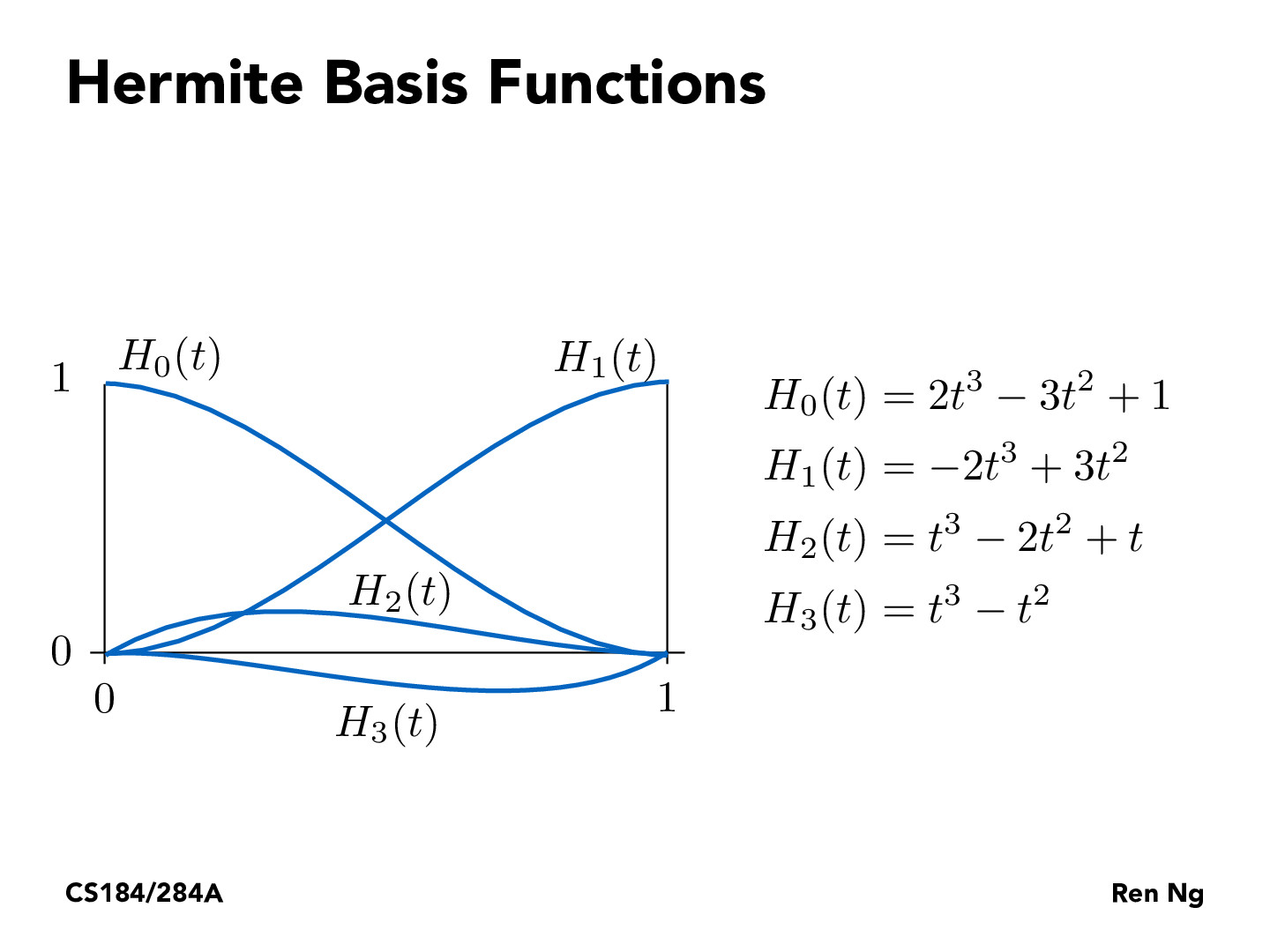Lecture 7: Geometry and Curves (38)ziyaointl

Notice that H_0 starts off at 1, which corresponds to the fact that the first parameter to Hermite interpolation (P(0)) is the location of the first point. Similarly, H_1 ends at 1, and its parameter (P(1)) is the location of the second point. This way it is ensured that the end result goes through both points.alexkassil

Are these a basis for cubic polynomials between 0 and 1 or for all cubic polynomials? Is there any difference?jinwoopark1673

I believe if they are basis for [0,1], then it must also be the basis for the whole real range. We can tell from looking at lower exponents to higher ones to see that they must be linearly independent.jcebreros1

Following up on jinwoopark1673, I believe it is possible to interpolate to an arbitrary interval [x1,x2] by mapping that interval to the interval [0,1]henryee333

Extending ziyaointl's observation, notice that H_2 starts off with a derivative of 1 (H_2'(0) = 1) and whereas H_0, H_1, H_3 start with derivatives of 0 (H_0'(0) = H_1'(0) = H_3'(0) = 0) so that the parameter h3 = P'(0) determines the slope at x=0.